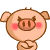﻿ 看《深度探索C++对象模型》，对于临时对象的疑问 | Code Bye

# 看《深度探索C++对象模型》，对于临时对象的疑问

5年前 (2017-04-11) 1206次浏览

//c++伪码
//T temp=a+b;
T temp;
temp.operator+(a,b); //(1)
//c=temp;
c.operator=(temp);//(2)
temp.T::~T();
（1）和（2）这两行假如是真实代码应该无法通过吧？本人在VC2015中试了一下是无法编译通过的，所以叫“伪码”？

2

2

5

5

temp.T::~T();
===================

4

```T temp = a + b;
//应该是
T temp(a + b);
//假如 operator+ 是成员重载那么就是 a.operator+(b)
T temp(operator+(a, b));```

```//假设有
typedef std::string T;
T a, b, c;
//那么
c = a + b;
//相当于
c.operator=(std::operator+(a, b));```

2

temp.operator+(a,b); 多了一个参数

c.operator=(temp);  等价于 c = temp;

1

T c=a+b; 调用的是构造函数

T c=a+b; 调用的是构造函数

T c=a+b; 调用的是构造函数40

VC调试时按Alt+8、Alt+7、Alt+6和Alt+5,打开汇编窗口、堆栈窗口、内存窗口和寄存器窗口看每句C对应的汇编、单步执行并观察相应堆栈、内存和寄存器变化，这样过一遍不就啥都明白了吗。

CodeBye 版权所有丨如未注明 , 均为原创丨本网站采用BY-NC-SA协议进行授权 , 转载请注明看《深度探索C++对象模型》，对于临时对象的疑问

[1034331897@qq.com]